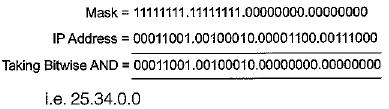Courses

## 15 Questions MCQ Test Mock Test Series - Computer Science Engg. (CSE) GATE 2020 | Introduction And IP Addressing (Advance Level) - 1

Description
QUESTION: 1

### To prevent signal alternation, what is the max number of repeaters that can be placed on one 10 Base 5 or 10.Base 2 network?

Solution:

Maximum 4 repeaters can be placed in one transmission path between two nodes while 5 between two nodes.

QUESTION: 2

### Which IP class provides the fewest numbers of hosts?

Solution:

Number of host in class A = 224 - 2 Number of host in class B = 216 - 2 Number of host in class C = 28 - 2 Number of host in class D = 0

QUESTION: 3

### A repeater takes a weak and or corrupted signal and _____ it.

Solution:

A repeater takes a weak or corrupted signal and regenerate it

QUESTION: 4

A subnet mask in class A can have ______ 1’s with the remaining bits 0’s.

Solution:

Default subnet mask of class A network 11111111-00000000-00000000-00000000
⇒ 255-0-0-0.

QUESTION: 5

To interconnect two IP classes, class A and class C networks

Solution:

Router is used to connect two different class networks.

QUESTION: 6

Given an IP address 156.233.42.56 with a subnet mask of 7 bits. How many hosts and subnets are possible.

Solution: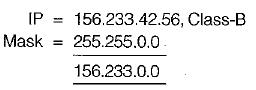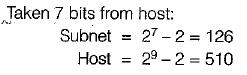QUESTION: 7

Class_______has the greatest number of hosts per given network address.

Solution:

Number of hosts in class A = 224 - 2 Number of hosts in class B = 216 - 2 Number of hosts in class C = 28 - 2

QUESTION: 8

The effective bandwidth is based on ______ .

Solution:

The effective data burst is a measure of average data rate, peak data rate and maximum data rate.

QUESTION: 9

Identify the unequal pair(s):

Solution:

QUESTION: 10

There are three IP addresses as given below:
X = 202.23.14.150
Y = 168.19.200.12
Z = 72.192.52.210

Which of the following statements is/are correct?

Solution:

In IP add re sse s class A, B, and C used for general purpose.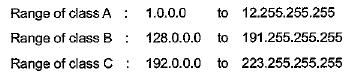QUESTION: 11

The default subnet mask for a Class B network can be

Solution:

Class A = 255.0.0.0
Class B = 255.255.0.0
Class C = 255.255.255.0

QUESTION: 12

Match List-I (Function) with List-11 (Layer) and select the correct answer using the codes given below the lists: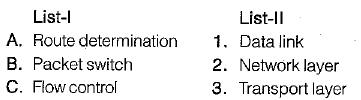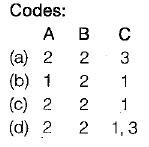Solution:

Packet switching→ network iayer.
Route determination→ 4 network layer.
Flow control → datalink and transport layer.

QUESTION: 13

Match List-I (Function) with List-lI (Layer) and select the correct answer using the codes given below the lists

List-I
A. Reassembly of packets
B. Responsibility for delivery between adjacent nodes
C. Mechanical electrical and functional interface
D. Error correction and retransmission

List-II
1. Transport
3. Physical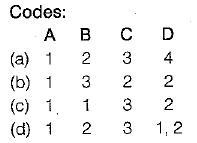Solution:
• Reassembly of packets is done at transport iayer.
• Responsibility for delivery between adjacent nodes is of data link layer.
• Mechanical electrical and functional interface is related to physical layer.
•  Error correction and retransmission is done at data link layer as well as transport layer.
QUESTION: 14

A subnet mask in class A has fourteen 1’s. How many subnet does it define?

Solution: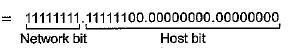6 bit are used for subnetting.
So number of possible subnets - 26 = 64.

QUESTION: 15

In a class A subnet, we know the IP address of one of the hosts and the mask as given below: